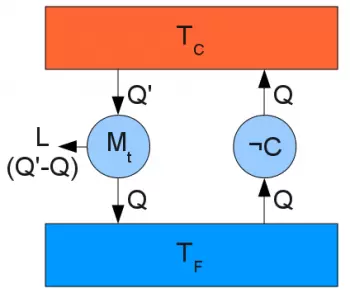# Second Law of ThermodynamicsThe second law of thermodynamics is a principle of classical thermodynamics that establishes the irreversibility of many thermodynamic events, such as the passage of heat from a hot body to a cold one. Unlike other laws of physics such as the law of universal gravitation or Maxwell's equations, the second principle is fundamentally linked to the arrow of time.

The second principle of thermodynamics has several equivalent formulations, one of which is based on the introduction of a state function, entropy: in this case the second principle states that the 'entropy of a system isolated from thermal equilibrium tends to to increase over time until balance is achieved. In statistical, classical and quantum mechanics, entropy is defined from the volume in the phase space occupied by the system to automatically satisfy (by construction) the second principle.

## Formulations of the Second Law of Thermodynamics

There are many equivalent formulations of this principle. Those that historically proved to be the most important are:

"It is impossible to perform a transformation whose only result is to transfer heat from a colder body to a warmer one without the contribution of external work" (Clausius' formulation).

"It is impossible to perform a cyclic transformation whose only result is the transformation in the work of all the heat absorbed by a homogeneous source" (formulation of Kelvin - Planck).

"It is impossible to make a thermal machine whose efficiency is 100%".

In modern physics, however, the most widely used formulation is that based on the entropy function:

"In an isolated system, entropy is a function that does not diminish with time"

This principle has had a significant impact from a historical point of view. In fact, it implicitly sanctions the impossibility of carrying out the so-called perpetual movement of the second species and by the irreversibility of thermodynamic processes an arrow of time is defined.

The two principles of macroscopic thermodynamics also apply to open systems, and are generalized through exergy.

### Equivalence of the First Two Declarations

The equivalence of the Kelvin-Planck sentence and the Clausius sentence can be shown by the following argument for the absurd.

In what follows, for reasons of brevity and clarity to be denoted by Kelvin the corresponding proposition enunciated Kelvin, with non-Kelvin his negation, with Clausius the corresponding proposition enunciated Clausius and with no Clausius his denial.

Kelvin implies Clausius

Schematization of a thermal machine based on an anti-Clausius machine, which violates Kelvin's statement

Suppose it is absurd that Clausius's claim is false, that is, that there is a cyclic refrigerating machine capable of transferring heat from a cold source to a hot one, without the contribution of external work.

Let Q be the quantity transferred for each cycle of the machine from the cold source to the hot one.

Then we can do a work of the thermal machine between the two sources, in such a way that it subtracts in each cycle a quantity of heat Q 'from the heat source, the transfer to the cold quantity QA and convert the difference Q' - Q at work.

The cold source then does not undergo any net transfer of heat and, therefore, our system of thermal machines is extracting heat, globally, only from the hot source, producing exclusively work, in violation of the Kelvin-Planck formulation of the second principle .

Clausius implies Kelvin

Schematization of a thermal machine based on an anti-Kelvin machine, which violates Clausius' statement

Now suppose that we can completely convert heat into work, extracted by a cyclic machine from a single source S at constant temperature.

Let L be such work extracted in a cycle.

Then we can take a second source S 'at a higher temperature and operate a refrigerating machine between the two sources, which absorbs the work produced by the other machine in each cycle.

Therefore, there is a net transfer of heat from the cold source S to the hot source S ', in violation of the Clausius declaration.

Author:

Published: May 17, 2018
Last review: May 17, 2018